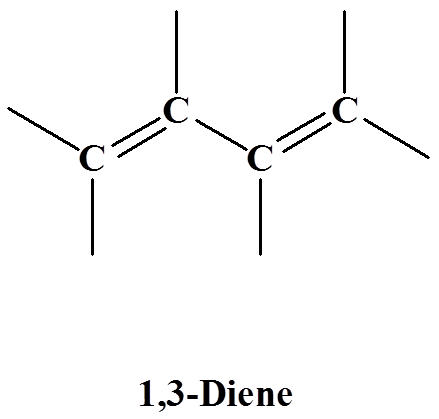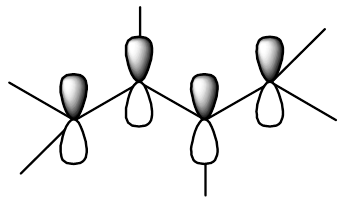# 15.1: Stability of Conjugated Dienes - Molecular Orbital Theory

$$\newcommand{\vecs}{\overset { \rightharpoonup} {\mathbf{#1}} }$$ $$\newcommand{\vecd}{\overset{-\!-\!\rightharpoonup}{\vphantom{a}\smash {#1}}}$$$$\newcommand{\id}{\mathrm{id}}$$ $$\newcommand{\Span}{\mathrm{span}}$$ $$\newcommand{\kernel}{\mathrm{null}\,}$$ $$\newcommand{\range}{\mathrm{range}\,}$$ $$\newcommand{\RealPart}{\mathrm{Re}}$$ $$\newcommand{\ImaginaryPart}{\mathrm{Im}}$$ $$\newcommand{\Argument}{\mathrm{Arg}}$$ $$\newcommand{\norm}{\| #1 \|}$$ $$\newcommand{\inner}{\langle #1, #2 \rangle}$$ $$\newcommand{\Span}{\mathrm{span}}$$ $$\newcommand{\id}{\mathrm{id}}$$ $$\newcommand{\Span}{\mathrm{span}}$$ $$\newcommand{\kernel}{\mathrm{null}\,}$$ $$\newcommand{\range}{\mathrm{range}\,}$$ $$\newcommand{\RealPart}{\mathrm{Re}}$$ $$\newcommand{\ImaginaryPart}{\mathrm{Im}}$$ $$\newcommand{\Argument}{\mathrm{Arg}}$$ $$\newcommand{\norm}{\| #1 \|}$$ $$\newcommand{\inner}{\langle #1, #2 \rangle}$$ $$\newcommand{\Span}{\mathrm{span}}$$$$\newcommand{\AA}{\unicode[.8,0]{x212B}}$$

## Introduction

Conjugated dienes are characterized by alternating carbon-carbon double bonds separated by carbon-carbon single bonds. Cumulated dienes are characterized by adjacent carbon-carbon double bonds. While conjugated dienes are energetically more stable than isolated double bonds. Cumulated double bonds are unstable. The chemistry of cumulated double bonds can be explored in advance organic chemistry courses. The chemistry of isolated alkenes is covered in Chapters 8 and 9 of this LibreText. The chemistry of conjugated double bonds is the focus of this chapter.## Conjugated Diene Stability

Conjugated dienes are more stable than non conjugated dienes (both isolated and cumulated) due to factors such as delocalization of charge through resonance and hybridization energy. This stability can be seen in the differences in the energies of hydrogenation between isolated and conjugated alkenes. Since the higher the heat of hydrogenation the less stable the compound, it is shown below that conjugated dienes (~54 kcal) have a lower heat of hydrogenation than their isolated (~60 kcal) and cumulated diene (~70 kcal) counterparts.

Here is an energy diagram comparing different types of bonds with their heats of hydrogenation to show relative stability of each molecule:## Stability of Conjugated Dienes - The Resonance Explanation

Conjugated double bonds are separated by a single bond. 1,3-dienes are an excellent example of a conjugated system. Each carbon in 1,3 dienes is sp2 hybridized and therefore has one p orbital. The four p orbitals in 1,3-butadiene overlap to form a conjugated system.The resonance structure shown below gives a good understanding of how the pi electrons are delocalized across the four carbons in this conjugated diene. This delocalization of electrons stablizes the conjugated diene:## Stability of Conjugated Dienes - The Molecular Orbitals Explanation

A molecular orbital model for 1,3-butadiene is shown below. Note that the lobes of the four p-orbital components in each pi-orbital are colored differently and carry a plus or minus sign. This distinction refers to different phases, defined by the mathematical wave equations for such orbitals. Regions in which adjacent orbital lobes undergo a phase change are called nodes. Orbital electron density is zero in such regions. Thus a single p-orbital has a node at the nucleus, and all the pi-orbitals shown here have a nodal plane that is defined by the atoms of the diene. This is the only nodal surface in the lowest energy pi-orbital, π1. Higher energy pi-orbitals have an increasing number of nodes. Since 1,3-butadiene has four pi electrons. The two bonding molecular orbitals are filled to explain the measurable stability of conjugated double bonds.Exercise

1. The heat of hydrogenation for allene is about 300 kJ/mol. Order a conjugated diene, a non-conjugated diene, and allene in increasing stability.